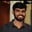Related Tags

python
communitycreator

# What are stacks in Python?Buchiredddypalli Koushik

A stack is a linear data structure that stores items in either the Last-In/First-Out (LIFO) or First-In/Last-Out (FILO) manner.

A stack is a limited access data structure, which means that elements can only be added and removed from the stack at the top. In other words, elements are added and removed from only one end of the stack.

To insert an element into a stack, we use the append() operation. To remove an element, we use the pop() operation.

Stacks in Python can be implemented in the following ways:

• list
• collections.deque
• queue.LifoQueue

### How to use list to implement stack

# Using List
stack=[]
# append() function to push an element into stack.

stack.append("A")
stack.append("B")
stack.append("C")
stack.append("D")
stack.append("E")
stack.append("F")
stack.append("G")

print("Initial Stack")
print(stack)

# pop() function to pop element from stack in LIFO order.
print("\nElements popped from stack :")
print(stack.pop())
print(stack.pop())
print(stack.pop())

print("\nFinal stack after the elements are popped : ")
print(stack)

In the program above, we first declare an empty stack. After that, we use the append() method to add elements into the stack. Later, we use the pop() method to delete the elements.

Finally, we print the stack, which is in LIFO order.

### How to implement stack with collections.deque

# Using Collections.deque

from collections import deque
stack=deque()
stack.append("DS Lab")
stack.append("Coding")
stack.append("DMS")
stack.append("Scc")
stack.append("Msmm")
print("Initial Stack :")
print(stack)
print("\nRemoval of Elements :")
print(stack.pop())
print(stack.pop())
print("\nFinal Stack")
print(stack)


In this program, we use the collections.deque module and add an element with the append() method. We also use the pop() method to delete an element.

### How to implement stack with the queue module

# Using Queue module
from queue import LifoQueue
stack=LifoQueue(maxsize=5)
# To know the size of elements in the stack we use the method q.size()
print(stack.qsize())
# Put function to append the elements into the stack
stack.put("AB")
stack.put("BC")
stack.put("CD")
stack.put("DE")
stack.put("EF")
print("Full : ",stack.full())
print("Size : ",stack.qsize())
# Get Function to pop the elements from the stack
print("\nElements Removed : ")
print(stack.get())
print(stack.get())
print("\nEmpty:",stack.empty())


In this program, we use the Queue module. To add elements, we use the put() method, and to remove/delete an element, we use the get() method. We also use the stack.full() method to check whether the stack is full or not.

RELATED TAGS

python
communitycreator

CONTRIBUTORBuchiredddypalli Koushik
RELATED COURSES

View all Courses

Keep Exploring

Learn in-demand tech skills in half the time